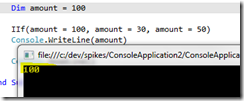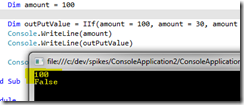# VB.NET and IIF-statements

The IIF-statements in VB.NET are a handy way to shorten our code and to make it more readable. It’s also an easy way to introduce subtle bugs into our systems.

Given the following code:

```        Dim amount = 100

IIf(amount = 100, amount = 30, amount = 50)
Console.WriteLine(amount)```

When run, the app will print out 30, yes? Well, actually no. Here’s the actual output when the app is executed:What happens is that the code inside the true-part of the IIF-statement is not an assignment.Instead, it’s interpreted by the compiler as aboolean expression. Now let’s try to modify our code so that it stores the return value of the IIF-statement in a variable:

```        Dim amount = 100

Dim outPutValue = IIf(amount = 100, amount = 30, amount = 50)
Console.WriteLine(amount)
Console.WriteLine(outPutValue)```

This is the output when executed:As you can see, the IIF statement returns False, because it compares the equality of variable “amount” to value 30.

To correct the above code one should change it to the following:

```        Dim amount = 100

amount = IIf(amount = 100, 30, 50)
Console.WriteLine(amount)```

Bottom line: IIF-statements in VB.NET shouldn’t be used for assignments.